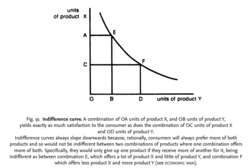# indifference curve

(redirected from Indifference curves)

## Indifference curve

The expression in a graph of a utility function, where the horizontal axis measures risk and the vertical axis measures expected return. The curve connects all portfolios with the same utility.

## Indifference Curve

A curve on a graph where the x-axis represents a quantity of one good and the y-axis represents a quantity of a second good where the curve represents the universe of quantities with the same utility for a rational investor. The indifference curve is convex, or roughly U-shaped.Fig. 91 Indifference curve. A combination of OA units of product X, and OB units of product Y, yields exactly as much satisfaction to the consumer as does the combination of OC units of product X and OD units of product Y.

Indifference curves always slope downwards because, rationally, consumers will always prefer more of both products and so would not be indifferent between two combinations of products where one combination offers more of both. Specifically, they would only give up one product if they receive more of another for it, being indifferent as between combination E, which offers a lot of product X and little of product Y, and combination F which offers less product X and more product Y (see ECONOMIC MAN).

## indifference curve

a curve showing alternative combinations of two products, each of which gives the same UTILITY, or satisfaction. See Fig. 91 . Indifference curves are used (along with BUDGET LINES) to determine a consumer's equilibrium purchases of two products and to analyse the effect of changes in the relative prices of these two products upon quantities demanded (see PRICE EFFECT). See CONSUMER EQUILIBRIUM, INDIFFERENCE MAP.
Collins Dictionary of Economics, 4th ed. © C. Pass, B. Lowes, L. Davies 2005
References in periodicals archive ?
This is based on the economic theory of choice, where an investor chooses among the opportunities by specifying a series of curves that are called indifference curves. These so-called curves are constructed so that everywhere, along the same curve, the investor is assumed to be equally satisfied.
Technical rate of substitution in consumption between X and R, reflected by the shape or the slope of indifference curves, provides additional information about an individual's preference for one good or another.
(8) The top panel shows households preferences represented by indifference curves over price values, q, and amounts of assets for period 2, [a.sub.2].
As we wish to analyze insurance demand graphically, we particularly investigate the properties of PT's indifference curves in two-outcome diagrams.
One way to assess the degree of inclusive growth of a country is to use indifference curves in which the horizontal axis shows the population arranged in ascending order of income (with the leftmost being the bottom 20% and rightmost the top 20%) and the y-axis shows the mean income of each corresponding income group.
For that purpose, he borrowed the concept of indifference curves from two economists of his times, Vilfredo Pareto (1848-1923) and Francis Y.
(12) The indifference curves are monotone (over the range of interest at least), and reflect the fact that a household can, in principle, receive additional consumption today in two ways: hold b fixed, as long as it faces a higher q, or increase borrowing b, but accept that the discount q will fall as default risk rises (though not so rapidly that q(b)b, which is what the household receives, falls).
As depicted in Conrow (1995), this can be illustrated graphically using government and contractor indifference curves sketched in cost-performance space.
Indifference curves express consumer's preferences of two kinds of goods.
The stability of preferences is further discussed in Section 4, while Section 5 briefly examines the implications of transitivity for indifference curves. Section 6 concludes the paper.
According to Figure 1, a series of indifference curves corresponding to each level of utility can be mapped, in which the indifference curve that has the longest distance with the origin.

Site: Follow: Share:
Open / Close